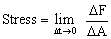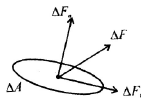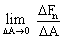# Physics Assignment Help With The Stress

1. Home
2. Physics
3. Matter Properties
4. The Stress

## 9.2 The Stress

To oppose the deformation caused by the external applied forces, the intensity of the internal force developed at a point to keep it in equilibrium was defined as stress and mathematically it is given by an expressionwhere DF is the internal restoring force acting on an small area DA.

The normal component of DF cause axial stress or normal stress while tangential component DF causes shear stress or tangential stress.In figure DF force is acting as an element DA which has normal and tangential component as Fn and Ft respectively. Thus, axial stress on the element isand shear stress as the element isThe axial stress either create tension in solid body or create compression in it. In former case, it is called tensile stress and in later one. It is called compressive stress. While the shear stress cause the layer of surfaces to slide over the lower one along the direction of shear stressing force.

(i) When the stress is applied tangential to a surface, it is called tangential or shearing stress.

(ii) Dimensional formula of stress is [ML–2T–2] and CGS and MKS units are dyne/cm2 and newton/m2 respectively.

(iii) Stress is a tensor quantity.

(iv) Through both stress and pressure and defined as force per unit area but even then they differ from each other due to following reasons:

(a) Pressure is always normal to the area while stress can be either normal a tangential.

(b) Pressure on a body is always compressive while stress can be either compressive or tensile.

(c) Pressure is a scalar while stress is a tensor. Shearing stress is exhibited by solids only as these have definite shape.### Email Based Assignment Help in The Stress

We are the leading online Assignment Help provider. Find answers to all of your doubts regarding the The Stress. Assignmenthelp.net provide homework, Assignment Help to the school, college or university level students. Our expert online tutors are available to help you in The Stress. Our service is focused on: time delivery, superior quality, creativity and originality.